# Solution assignment 08 Optimizing f(x)

### Assignment 8

Verify whether the function: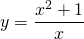has a maximum or a minimum.

### Solution

Naturally we have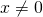.

Candidates for a maximum or a minimum can be found by making the first derivative equal to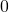and solve the resulting equation. We find the derivative using the quotient rule: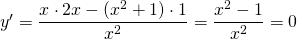A fraction equalsif its numerator equalsand thus we have to solve: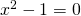and thus: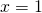or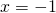In order to investigate whether a point is a maximum or a minimum we could use the second derivative. However this requires a lot of calculations. It is easier to consider the sign of the first derivative. The denominator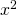is always positive, we only have to look at the numerator. This contains a quadratic function of which the graph is an 'opens up' parabola. Around the left intersection pointthe first derivative goes from + viato - and thus there is a maximum for. Forthe reverse holds and thus we have a minimum for this point.
The extremes are thus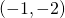(maximum) and(minimum).
This result seems strange: a minimum that is greater than a maximum but it is not. Look at the graph below. Notice that the line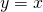is an asymptote.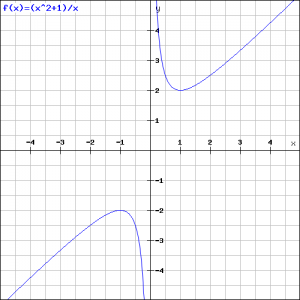There is also another approach. Before differentiating the function we first simplify it by dividing both numerator and denominator by.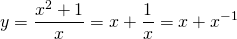This function is easier to handle and it immediately shows the existence of the asymptote.

0